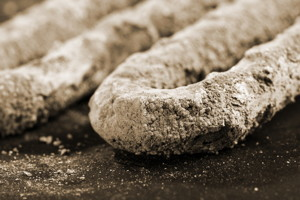# Examining Scaling Indices: What are They?

Virtually all natural water supplies, with the exception of rainwater and seawater, are saturated in calcium carbonate.#### By Paul Puckorius and Peter S. CartwrightMacro of calcium scum on electric heater. Image courtesy of Shutterstock.
Virtually all natural water supplies, with the exception of rainwater and seawater, are saturated in calcium carbonate. This is because the concentration of this compound, above which it becomes insoluble, is only about 10 mg/L, and these natural supplies usually contain that much. The whitish-gray deposit around faucet aerators, shower heads, tea kettles, etc., with which we're all very familiar, is mostly calcium carbonate scale.

Three mathematical models have been developed to predict the tendency of a particular water supply to form calcium carbonate scale. These are known as:

• The Langelier-Saturation Index (LSI)
• The Ryzner Stability Index (RSI)
• The Puckorius Scaling Index (PSI)

All are based on the pH of the saturation of calcium carbonate and use the following analytical values:

• Calcium hardness (expressed as CaCO3)
• Total alkalinity (expressed as CaCO3)
• Total dissolved solids (TDS)
• Temperature (°C)

The indices are calculated as follows:

1) LSI = pH - pHs, where pH is the actual pH, and pHs is the pH at saturation. pHs is calculated as follows:If LSI is positive, calcium carbonate scale may form; if LSI is negative, the water will dissolve scale. As with pH, these numbers are Logarithmic: every unit is a factor of 10.

2) RSI = 2 (pHs) -pH, where (as with LSI) pHs is the pH at saturation and pH is the actual pH. For RSI values below 6, the scaling tendency increases, and for values above, the water will dissolve scale. As with pH (and LSI), RSI values are factors of 10.

3) PSI = 2 (pHs) - pHeq, where pHs is the pH at saturation and pHeq is an equilibrium pH based on the total alkalinity value. As with RSI, if the PSI value is below 6, calcium carbonate scaling is predicted, and above 6, the water will dissolve scale. The PSI numbers are also factors of 10.

 Three IndicesThe LSI and RSI values are based on the actual pH values resulting from carbonate or bicarbonate concentration only; however, buffering agents such as caustic or ammonia can raise pH and indicate a false scaling tendency.

The PSI is designed to minimize the effects of buffering agents by developing an "equilibrium pHeq" from the alkalinity of the water.

 The three scaling indices are compared in the following examples taken from actual cooling water applications.Note that both the LSI and RSI predict scale formation while the PSI does not. In reality, no scaling occurred in these cooling towers; therefore, no scale inhibitor chemicals were required.

These calculations specifically address calcium carbonate formation, they can apply to any water use, but are not applicable to other scale-forming chemistries, such as calcium sulfate, calcium phosphate or various silica scales.

 pHs for PSI calculations as a function of total alkalinity.Likewise, LSI and RSI are also ineffective with these other chemistries.

About the Authors: Paul Puckorius is President and CEO of Puckorius & Associates. He has over 50 years experience in cooling water, boiler water and reuse water technology, specializing in corrosion, scale and microbiological problem solving, treatment selection and system start-ups. Peter Cartwright has had his own consulting engineering firm since 1980. He has a degree in Chemical Engineering from the University of Minnesota and is a registered Professional Engineer in that state.

More in Industrial